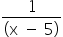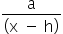Mathematics
Easy

Question

# What are the equations of the asymptotes of the function f(x) =+ 3 ?

## Horizontal: y = 5 , Vertical : x = 3Horizontal: y = -3 , Vertical : x = 5Horizontal: x = 5 , Vertical : y = -3Horizontal: x = -3 , Vertical : y = 5Hint:

## The correct answer is: Horizontal: y = -3 , Vertical : x = 5

### Here, we have to find the asymptotes to the graph y = 1/(x-5) +3.For the function, f(x) =+ kHorizontal asymptote: y = k Vertical asymptote: x = h In this case, asymptotes are x = 5 and y = -3.Hence, the correct option is B.

Horizontal asymptote is y = k and vertical asymptote is x = h, where (h,k) is a moving point on the graph.

### Related Questions to study#### With Turito Foundation.#### Get an Expert Advice From Turito.